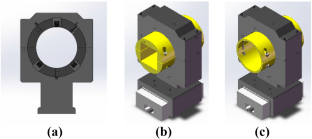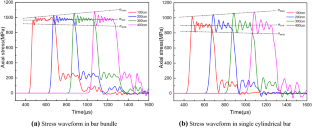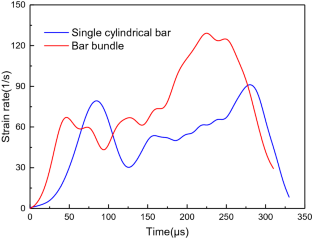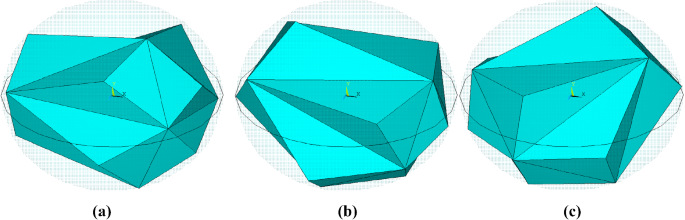# Research of an SHPB Device in Two-by-Two Form for Impact Experiments of Concrete-Like Heterogeneous Materials

## Abstract

A split Hopkinson pressure bar (SHPB) device in two-by-two form, including the bar bundle form and the single cylindrical bar form, was designed in response to the demand for the dynamic mechanical experiments for brittle materials such as concrete, rock, etc. The stress waveforms generated through a projectile impacting two different types of incident bars have been studied based on the one-dimensional stress wave theory and numerical simulation method. At last, based on the established two types of mesoscale concrete models with random convex polyhedral aggregates, we performed comparison analysis of SHPB numerical simulations for concrete materials with bar bundle and single cylindrical bar separately, so as to provide technical support for the manufacture and development of this experimental device. The results showed that the introduced two-by-two form SHPB device expanded the scope of practical application, and the wave dispersion effect existing in a large-diameter bar can be evidently reduced when we employed the bar bundle form, indicating its applicability to the dynamic mechanical experiments of concrete-like heterogeneous materials.

This is a preview of subscription content, access via your institution.Fig. 1Fig. 2Fig. 3Fig. 4Fig. 5Fig. 6Fig. 7Fig. 8Fig. 9Fig. 10Fig. 11Fig. 12Fig. 13Fig. 14Fig. 15Fig. 16Fig. 17Fig. 18Fig. 19Fig. 20Fig. 21Fig. 22

## References

1. 1.

Cady CM, Gray GT, Liu C, et al. Compressive properties of a closed-cell aluminum foam as a function of strain rate and temperature. Mater Sci Eng, A. 2009;525:1–6.

2. 2.

Aldoshan A, Mondal DP, Khanna S. High strain rate behavior of carbon nanotubes reinforced aluminum foams. J Eng Mater Technol. 2018;140:011011.

3. 3.

Xiong B, Demartino C, Xiao Y. High-strain rate compressive behavior of CFRP confined concrete: Large diameter SHPB tests. Constr Build Mater. 2019;201:484–501.

4. 4.

Lee S, Kim KM, Park J, et al. Pure rate effect on the concrete compressive strength in the split Hopkinson pressure bar test. Int J Impact Eng. 2018;113:191–202.

5. 5.

Ross CA, Tedesco JW, Kuennen ST. Effects of strain rate on concrete strength. Mater J. 1995;92:37–47.

6. 6.

Tang TX, Malvern LE, Jenkins DA. Dynamic compressive testing of concrete and mortar Engineering Mechanics in Civil Engineering. ASCE. 1984;663–666.

7. 7.

Malvern LE, Jenkins DA, Tang T et al. Dynamic compressive testing of concrete. 2nd Symposium on The Interaction of Non-Nuclear Munitions with Structures, Engineering and Services Laboratory-Tyndall AFB, Panama City Beach, FL 1985;194-199.

8. 8.

Malvern LE, Ross CA. Dynamic Response of Concrete and Concrete Structures. Florida Univ Gainesville, Dept of Engineering Sciences 1986.

9. 9.

Malvern LE, Jenkins DA, Jerome E et al. Dispersion correction for split-Hopkinson pressure bar data. Florida Univ Gainesville, Dept of Engineering Sciences, 1988.

10. 10.

Malvern LE, Jenkins DA. Dynamic testing of laterally confined concrete. California Inst of Tech Pasadena Dept of Information Sciences 1990.

11. 11.

Liu P, Hu D, Wu Q, et al. Sensitivity and uncertainty analysis of interfacial effect in SHPB tests for concrete-like materials. Constr Build Mater. 2018;163:414–27.

12. 12.

Ye ZB, Huang RY, Li YC, et al. Steel fiber-reinforced concrete under impact loading dynamic constitutive equation. Constr Build Mater. 2018;190:1049–55.

13. 13.

Chen JY, Zhang ZX, Dong HW, et al. Experimental study on dynamic damage evolution of concrete under multi-axial stresses. Eng Fail Anal. 2011;18:1784–90.

14. 14.

Albertini C, Montagnani M. Study of the true tensile stress-strain diagram of plain concrete with real size aggregate; need for and design of a large Hopkinson bar bundle. J Phys IV. 1994;4:C4-113–8.

15. 15.

Cadoni E, Labibes K, Berra M, et al. High-strain-rate tensile behaviour of concrete. Mag Concr Res. 2000;52:365–70.

16. 16.

Ning JG, Zhou FH, Wang ZH, et al. Constitutive model, failure mechanism and numerical method for reinforced concrete under intensive impact loading. Sci Sin Technol. 2016;46:323–31.

17. 17.

Guo RQ, Ren HQ, Zhang L, et al. Research progress of large-diameter split Hopkinson bar experimental technique. Acta Armamentarii. 2019;40:1518–36.

18. 18.

Wang LL. Foundations of stress waves. Dordrecht: Elsevier; 2011.

19. 19.

Wang J, Ren H, Wu X, et al. Blast response of polymer-retrofitted masonry unit walls. Compos B Eng. 2017;128:174–81.

20. 20.

Lv TH, Chen XW, Chen G. The 3D meso-scale model and numerical tests of split Hopkinson pressure bar of concrete specimen. Constr Build Mater. 2018;160:744–64.

21. 21.

Zhou Z, Li X, Liu A, et al. Stress uniformity of split Hopkinson pressure bar under half-sine wave loads. Int J Rock Mech Min Sci. 2011;48:697–701.

22. 22.

Xu W, Han Z, Tao L, et al. Random non-convex particle model for the fraction of interfacial transition zones (ITZs) in fully-graded concrete. Powder Technol. 2018;323:301–9.

23. 23.

Sheng P, Zhang J, Ji Z. An advanced 3D modeling method for concrete-like particle-reinforced composites with high volume fraction of randomly distributed particles. Compos Sci Technol. 2016;134:26–35.

24. 24.

Nitka M, Tejchman J. A three-dimensional meso-scale approach to concrete fracture based on combined DEM with X-ray $$\mu$$CT images. Cem Concr Res. 2018;107:11–29.

25. 25.

Zhang J, Wang Z, Yang H, et al. 3D meso-scale modeling of reinforcement concrete with high volume fraction of randomly distributed aggregates. Constr Build Mater. 2018;164:350–61.

26. 26.

Li X, Xu Y, Chen S. Computational homogenization of effective permeability in three-phase mesoscale concrete. Constr Build Mater. 2016;121:100–11.

27. 27.

Holmquist TJ, Johnson GR, Cook WH. A computational constitutive model for concrete subjected to large strains, high strain rates, and high pressures The 14th International Symposium on Ballistics. Qucbec. 1993;591–600.

28. 28.

Liu HF, Han L. Numerical simulation research on dynamic mechanical behaviors of concrete subjected to impact loading. Chin J Solid Mech. 2015;36:145–53.

29. 29.

Fang Q, Kong ZX, Wu H, et al. Determination of Holmquist-Johnson- Cook constitutive model parameters of rock. Eng Mech. 2014;31:197–204.

30. 30.

Diamond S, Huang J. The interfacial transition zone reality or myth. Proc Int RILEM Conf Lond & Spon. 1998;39:3.

31. 31.

Hao YF, Zhang XH, Hao H. Numerical analysis of concrete material properties at high strain rate under direct tension. Proc Eng. 2011;14:336–43.

32. 32.

Hao YF, Hao H, Li ZX. Numerical analysis of lateral inertial confinement effects on impact test of concrete compressive material properties. Int J Protective Struct. 2010;1:145–67.

33. 33.

Chen X, Wu S, Zhou J. Experimental and modeling study of dynamic mechanical properties of cement paste, mortar and concrete. Constr Build Mater. 2013;47:419–30.

34. 34.

Chen JK, Li JL, Zhu LM, et al. On the tension-induced microcracks’ nucleation in a PBX substitute material under impact compression loading. Int J Mech Sci. 2017;134:263–72.

35. 35.

Zencker U, Clos R. Limiting conditions for compression testing of flat specimens in the split Hopkinson pressure bar. Exp Mech. 1999;39:343–8.

36. 36.

Chen JQ, Wang H, Dan HC, et al. Random modeling of three-dimensional heterogeneous microstructure of asphalt concrete for mechanical analysis. J Eng Mech. 2018;144:04018083.

Download references

## Acknowledgements

This work was supported by the National Natural Science Foundation of China (Grant Nos. 51971188 and 51071134), the Science and Technology Major Project of Hunan Province (Grant No. 2019GK1012), and Huxiang High-Level Talent Gathering Program of Hunan Province-Innovative team (Grant No. 2019RS1059). All these supports are acknowledged.

## Author information

Authors

### Corresponding author

Correspondence to Zhilin Long.

## Appendix: Establishment of Mesoscale Aggregate Concrete Model and Generation of Random Convex Polyhedral Aggregate

### Appendix: Establishment of Mesoscale Aggregate Concrete Model and Generation of Random Convex Polyhedral Aggregate

The mesoscale aggregate concrete model with spherical aggregates is first introduced. Three mutually independent random numbers, i.e., $$rdm_{1}$$, $$rdm_{2}$$, and $$rdm_{3}$$ uniformly distributed between 0 and 1 are defined. In the three-dimensional Cartesian coordinate system, the center coordinates of the random spherical aggregate can be expressed as:

\begin{aligned} \left\{ {\begin{array}{l} x_{i} =X_{L} +R_{i} +(X_{R} -X_{L} -2\times R_{i} )\times rdm_{1} \\ y_{i} =Y_{B} +R_{i} +(Y_{T} -Y_{B} -2\times R_{i} )\times rdm_{2} \\ z_{i} =Z_{B} +R_{i} +(Z_{T} -Z_{B} -2\times R_{i} )\times rdm_{3} \\ \end{array}} \right. \end{aligned}
(A.1)

where $$R_{i}$$ is the radius of the generated aggregate, and $$X_{L}$$, $$X_{R}$$, $$Y_{B}$$, $$Y_{T}$$, $$Z_{B}$$, $$Z_{T}$$ are coordinates of the boundaries of the cuboidal specimen.

For the cylindrical specimen, Eq. (A.1) is corrected as:

\begin{aligned} \left\{ {\begin{array}{l} x_{i} =(R-R_{i} )\times rdm_{1} \times \cos (2\pi \times rdm_{2} ) \\ y_{i} =(R-R_{i} )\times rdm_{1} \times \sin (2\pi \times rdm_{2} ) \\ z_{i} =Z_{B} +R_{i} +(Z_{T} -Z_{B} -2\times R_{i} )\times rdm_{3} \\ \end{array}} \right. \end{aligned}
(A.2)Fig. 23

where $$R_{i}$$ is the radius of the cylindrical specimen, $$Z_{B}$$ and $$Z_{T}$$ are coordinates of the bottom and top boundaries of the cylindrical specimen, respectively. After the coordinates of the aggregate center are generated, the i-th aggregate to be placed must have no overlap with the previous (i-1)-th    one, that is, the distance between two ceners is greater than the sum of the aggregates’ radii, which can be expressed as:

\begin{aligned} \sqrt{(x_{i} -x_{n} )^{2}+(y_{i} -y_{n} )^{2}+(z_{i} -z_{n} )^{2}} \ge R_{i} +R_{n} , \quad n=1,2,\cdot \cdot \cdot \text{, }i-1 \end{aligned}
(A.3)

In this paper, we adopt a relatively simplified method to guarantee that the polyhedra have no overlaps with others, that is, to generate the polyhedral aggregates randomly within a spherical aggregate. First of all, a basic octahedral aggregate is generated randomly within a sphere, as shown in Fig. 23. Then, a method referred to as the “outer radial extension method” is utilized to extend the eight faces of the basic aggregate for one time, that is, to define a spatial straight line through the spherical center (abc) and the triangular surface centroid $$({x}',{y}',{z}')$$:

\begin{aligned} \left\{ {\begin{array}{l} x={x}'+({x}'-a)t \\ y={y}'+({y}'-b)t \\ z={z}'+({z}'-c)t \\ \end{array}} \right. \end{aligned}
(A.4)

Substituting Eq. (A.4) into the spherical equation $$(x-a)^{2}+(y-b)^{2}+(z-c)^{2}=r^{2}$$, we have

\begin{aligned} \left\{ {\begin{array}{l} t_{1} =-1+\frac{r}{\sqrt{({x}'-a)^{2}+({y}'-b)^{2}+({z}'-c)^{2}} } \\ t_{2} =-1-\frac{r}{\sqrt{({x}'-a)^{2}+({y}'-b)^{2}+({z}'-c)^{2}} } \\ \end{array}} \right. \end{aligned}
(A.5)

Set $$t>0$$, so the intersection point will be on the top of the extended surface. Connect the intersection point with the corresponding three vertices of the triangular surface, constituting the concave-convex irregular polyhedra, as shown in Fig. 24. After that, the convex polyhedral aggregate is generated by the connection of the eight salient points, as shown in Fig. 25. Finally, we can scale the aggregate down to 90% and perform Boolean operation in ANSYS software to generate the interfacial layer, as shown in Fig. 26. However, to reduce the amount of mesh cells and shorten calculation time, we adopt a rather simplified method dealing with the interfacial layer in this paper.Fig. 24Fig. 25Fig. 26

## Rights and permissions

Reprints and Permissions